## research papersJOURNAL OFSYNCHROTRONRADIATION
ISSN: 1600-5775
Volume 26| Part 1| January 2019| Pages 11-17

## Systematic study of a corrugated waveguide as a microwave undulator

aDepartment of Physics, SUPA, University of Strathclyde, Glasgow G4 0NG, UK, bThe Cockcroft Institute, Sci-Tech Daresbury, Keckwick Lane, Daresbury, Warrington WA4 4AD, UK, and cASTeC, STFC Daresbury Laboratory, Sci-Tech Daresbury, Keckwick Lane, Daresbury, Warrington WA4 4AD, UK
*Correspondence e-mail: liang.zhang@strath.ac.uk

(Received 2 April 2018; accepted 10 October 2018)

Microwave undulators have great potential to be used in short-wavelength free-electron lasers. In this paper, the properties of a corrugated waveguide and its performance as an undulator cavity for a UK X-ray free-electron laser were systematically studied. The equations presented in this paper allow a fast estimation of the dimensions of the corrugated waveguide. An undulator cavity operating at 36 GHz designed for the HE11 and HE12 modes was investigated and the performance of both modes compared.

### 1. Introduction

Undulators are one of the most important components in a free-electron laser (FEL) (Deacon et al., 1977; Huang & Kim, 2007). Currently, the conventional permanent magnet undulator (PMU) plays the dominant role. Microwave undulators (MUs) (Shintake et al., 1982, 1983) that also have a periodic magnetic field can be potential undulators and have the following advantages. (i) Fast dynamic control of the polarization (Shumail & Tantawi, 2016). (ii) Easy control of the field strength, which can be adjusted through the input microwave power, whereas, in a PMU, mechanical methods to adjust the magnet gap or the magnet period have to be used which can be complicated. (iii) It is challenging for a PMU to achieve short periods as the magnetic field strength would be significantly reduced. In contrast, for an MU the equivalent period is mainly determined by the wavelength of the electromagnetic wave, therefore a short period can be achieved if the MU operates at a higher frequency. (iv) The MU is essentially a metallic cavity and hence it is robust against damage by ionizing radiation near electron beam dump regions, as compared with the PMUs that are made of rare-earth materials, which may be more susceptible to damage in harsh ionizing radiation environments.

However, since the concept of the microwave undulator was proposed in 1982 (Shintake et al., 1982), progress has taken longer than expected, mainly due to the limited availability of high-power microwave sources. In 1983 (Shintake et al., 1983), the first MU experiment was carried out and an equivalent magnetic field Bu of 0.045 T with an undulator parameter K of 0.24 was achieved when driven by a 300 kW, 2.856 GHz microwave source. A ridged rectangular cavity was used and a quality factor Q of 7100 was measured. To achieve a similar performance to a state-of-the-art PMU, for example, 1.29 T for a 15 mm-period PMU used in the Swiss-FEL at the Paul Scherrer Institute, the required driving power would need to be more than 20 MW at 10.5 GHz (assuming the same Q factor of 7100 can be achieved for a similar structure scaled for operation at 10.5 GHz). However, the electric field at the wall of the ridged rectangular cavity would be too high and susceptible to microwave breakdown. A significant improvement on the MU was made with the use of a low-loss HE11 mode in a corrugated waveguide. A cavity made of a corrugated waveguide operating at the X-band was measured and it was able to achieve a Q factor as high as 91 000. When it was driven by a 50 MW SLAC klystron at 11.424 GHz (Tantawi et al., 2014), such an MU was able to achieve an equivalent Bu of 0.65 T with a period of 13.9 mm.

The corrugated waveguide is normally overmoded. The numerical calculation of the eigenmodes of the cavity made with the corrugated waveguide is time-consuming. In this paper, analytical equations and design constraints are summarized which could be used to quickly estimate the dimensions of the structure. The microwave undulator can be operated at a high millimetre-wave frequency to achieve a smaller period. In this paper, a corrugated waveguide operating at 36 GHz was designed as an MU for a UK XFEL.

The paper is organized as follows. In Section 2, the principle of the MU and the relation between the cavity and the microwave sources is presented. Section 3introduces the theory of the corrugated waveguide and the equations that determine the dimensions, field distribution and the loss coefficient. Section 4details the simulation results of an MU operating at 36 GHz. Both the HE11 and HE12 operating modes were investigated and their performances compared.

### 2. Principles of MU

The principle of the MU can be found in the literature (Pellegrini, 2006; Seidel, 2001; Shintake et al., 1983; Shumail & Tantawi, 2016). The relativistic electrons in an MU cavity will interact with both the electric field Ex = E0 sin(2πz/λg) sin(ωt) and magnetic field By = B0 cos(2πz/λg) cos(ωt). Compared with a PMU, the Lorentz force in an MU can be rewritten in the form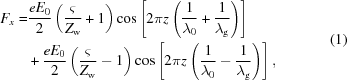where e is the charge on the electron, λ0 is the free space wavelength, and λg is the wavelength of the electromagnetic wave in the undulator cavity. E0 and B0 are the peak electric and magnetic field strength in the microwave undulator cavity, respectively. Zw is the wave impedance in the cavity and ς is the wave impedance in free space. The second term leads to a long wavelength and is undesirable in the undulator of a short-wavelength FEL. The second term can be ignored if the wave impedance is close to the free-space impedance, which means the operating frequency is far from the cut-off frequency of the waveguide. In this case, the equivalent magnetic field Bu and wavelength λu of the microwave undulator are given by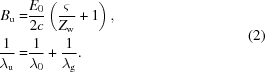If the microwave source starts to fill the cavity at time zero, the stored energy at time t in the cavity can be expressed as (Alvarez, 1986)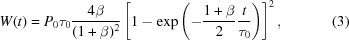where P0 is the input power, τ0 = Q0/ω, and β = Q0/Qe. Q0 and Qe represent the intrinsic and external quality factor of the cavity. In a steady state, where tτ0, the stored energy reaches its maximum value of P0τ0 if the coupling aperture is specifically designed to achieve β = 1. The input power in this case will be equal to the Ohmic loss in the cavity. In the cases of an under-coupled (β < 1) and over-coupled (β > 1) regime, the stored energy in the steady state is smaller. The filling time of the cavity ttf can be calculated if a charging factor η, defined as the ratio of the charged energy and its maximum value, is known,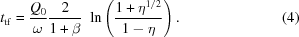From equations (3) and (4), to achieve maximum storage at a given input power, a higher Q factor of the cavity is preferred; however, the filling time increases simultaneously. In applications of MUs, high-power microwave sources with output powers of megawatts or more are needed to achieve a high equivalent magnetic field. Such high-power microwave sources normally operate in pulsed mode to reduce the power supply requirements and associated thermal stress. The filling time of the MU cavity should match the pulse length of the microwave source. The coupler can be slightly over-coupled to reduce the filling time while maintaining a high Q factor. If the input microwave frequency is 36 GHz, with a 2 µs pulse length, the Q factor of the cavity should be under 150 000 if β = 1 and η = 0.9.

The electric field strength inside the cavity along the electron beam path can be calculated by careful selection of the operating mode of the cavity. Knowing the input power and Q factor, the parameters of the MU can be determined.

### 3. Corrugated waveguide

A high-Q low-loss cavity is of great importance when it is used as an MU. In a circular waveguide, the mode with the lowest loss is the TE01 mode. However, the field strength at the waveguide center for this mode is small and hence is not a good option for an MU as the electrons are to propagate down the center of the structure. A corrugated waveguide, as shown in Fig. 1, can be used in other applications such as a feed horn for a transmission line system due to its advantages of low cross-polarization field, low loss and wide bandwidth. Its low attenuation feature is attractive for the transmission of high-power microwaves. The fundamental mode HE11 was found to have lower loss compared with the TE01 mode in the circular waveguide. It has been used to transport megawatt-level millimetre-wavelength radiation generated by gyrotron oscillators for fusion experiments such as ITER. In recent years, the corrugated waveguide has been proposed as an MU (Shumail et al., 2011; Chang et al., 2012; Toufexis & Tantawi, 2017).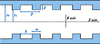Figure 1 Schematic drawing of the corrugated waveguide.

The corrugated waveguide contains circular waveguide steps and smooth sections. The properties of a corrugated waveguide with arbitrary radial corrugation depth can be accurately solved using a mode-matching method (James, 1981; Neilson et al., 1989; Zhang et al., 2016). For the periodically corrugated waveguide used for transmitting the microwave power, analytical equations can be derived based on the fact that the waveguide radius is larger than the wavelength. The propagation characteristics of the periodically corrugated waveguide were studied using the simplified surface-impedance approach or the rigid equations taking into account the spatial harmonics in the corrugation gaps (Clarricoats & Olver, 1984; Dragone, 1980; Clarricoats & Saha, 1971; Kowalski et al., 2010).

The surface-impedance approach assumes that only the lowest transverse magnetic (TM) standing wave exists in the slot and ignores its spatial harmonics. It gives a good approximation when the period per wavelength, defined by λ0/p, is a reasonably large value and the corrugation slot length, defined by w = pb in Fig. 1, is a small value. At low frequency, for example in the application of the microwave undulator, these assumptions would normally be satisfied. If only the lowest TM standing wave were present in the corrugation slot, its surface admittance at r = r1 can be written as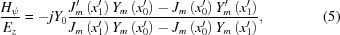where m indicates the azimuthal mode number, x1′ = kr1 and x0′ = kr0. Jm and Ym are the first and second kind of Bessel functions of order m, respectively. Y0 is the free-space wave admittance. The surface admittance becomes 0 when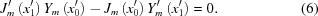This is known as the balanced hybrid condition. If the operating frequency (f) and the waveguide radius (either r0 or r1) are given, the corrugation depth d = r0r1 can be determined from the equation. At m = 1 and x1′ ≫ 1, the surface admittance can be further simplified as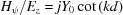. The corrugation depth d would be equal to λ0/4 to ensure Hψ = 0.

Under the balanced hybrid condition and x1′ ≫ 1, the dispersion curve between k and kz is determined by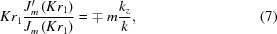where K2 = k2kz2, the −' in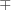' denotes the HE modes, and the +' denotes the EH modes. For a large radius, which leads to kzk and m = 1, equation (7)can be further simplified to J0(Kr1) = 0 for HE1n modes and J2(Kr1) = 0 for EH1n modes (Shumail, 2014).

To describe the field distribution inside the corrugated waveguide, different eigenmode sets using TE/TM and HE/EH combinations have been derived (Crenn & Charollais, 1996). The field inside the corrugated waveguide with the linearly polarized mode sets can be simplified as (Clarricoats & Saha, 1971)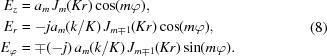Usually, a higher order HE or EH mode is not linearly polarized. The suitable operating modes in the corrugated waveguide for the microwave undulator application are the HE11 and HE12 modes because they are low loss, linearly polarized and have the peak electric field at the waveguide center. The electric field patterns of the HE11 and HE12 modes are shown in Fig. 2. The HE12 mode has larger field density at the waveguide center. It can have a bigger electric field compared with the HE11 mode at the same input power.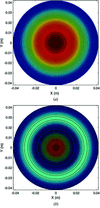Figure 2 Contour plot of the electric field patterns of the (a) HE11 and (b) HE12 modes.

The attenuation coefficient defined by the ratio between the lost power and the transported power per meter for the HE1n modes, under the balanced hybrid condition, can be written as (Clarricoats & Olver, 1984; Doane, 1985)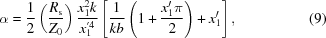where Z0 = 1/Y0 is the free space wave impedance, Rs = (πfμ0σ)1/2 is the resistivity of the corrugated metal waveguide and σ is the conductivity of the metal. In this paper, oxygen-free high-conductivity copper was chosen and σ = 5.8 × 107 S m−1 was used in the simulation. Equation (9) implies that the attenuation coefficient is proportional to r1−3 and f−2 in the case of x1′ ≫ 1.

The corrugated waveguide can be shortened at both ends to form a cavity. The resonance frequency of the corrugated waveguide can be estimated as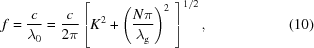where c is the speed of light, N is an integer and λg is the wavelength of the resonance mode in the cavity. Because the corrugated waveguide is normally overmoded, λ0λg when N = 2. As mentioned earlier, the surface-impedance approach gives a good approximation when λ0/p is a reasonably large value. Therefore, when designing the corrugated waveguide, the period p can be selected to be smaller than λ0/2. It is of course the case that the smaller the period, the better'; however, as the operating frequency increases, the wavelength becomes smaller, resulting in a small corrugation period and a thin corrugation slot of w ≃ 0 which significantly increases the machining difficulty. The final choice of the geometry should therefore also consider the machining tolerance.

Since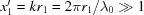has to be satisfied, the corrugated waveguide radius r1 is a large value. As the attenuation coefficient is proportional to r1−3, it is preferable to have a large r1 at a given operating frequency. From equation (8), the waveguide radius affects the field strength if the input power is a fixed value. In a microwave undulator, a high field at the electron beam path, in this case the waveguide center, is desired. Therefore r1 should be as small as possible under the constraint of kr1 ≫ 1. On the other hand, it is preferred for the electron beam that travels through the microwave undulator to see a uniform field in the radial direction. The minimum waveguide radius can be solved from the field pattern of the operating mode if the electron beam aperture Rb and a threshold, for example 90% of the maximum field at the beam edge, are defined. For HE1n modes, it follows that J1(KRb) = 0.9. Taking the first two solutions of J0(Kr1) = 0, K is approximately equal to 2.4/r1 or 5.5/r1 for the HE11 or the HE12 mode, respectively. Therefore the following parameters were chosen,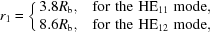resulting in a reasonable value of r1. Meanwhile the value of r1 should satisfy x1′ = 2πr1/λ0 ≫ 1. If x1′ = 5 is used, then r1 ≥ 0.8λ0 and the results become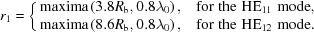### 4. Design of a corrugated waveguide for an MU

A corrugated waveguide cavity operating at 36 GHz was designed as a potential microwave undulator for the UK XFEL. The initial geometry parameters were calculated from the equations described in previous sections. Both of the HE11 and HE12 modes are considered. A summary of the geometry parameters as well as the undulator deflection parameter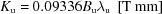are listed in Table 1.

 Table 1Parameters of the MU composed of a corrugated waveguide
 Operating mode HE11 HE12 Operating frequency (GHz) 36 36 λ0 (mm) 8.33 8.33 Rb (mm) 2.0 2.0 r1 (mm) 4Rb = 8.0 9Rb = 18.0 d = λ0/4 (mm) 2.1 2.1 λg (mm) 9.06 9.12 p = λg/3 (mm) 3.00 3.02 w (mm) 0.5 0.5 b = p − w (mm) 2.50 2.52 Q factor 94344 187073 Input power (MW) 50 50 Peak Ex on axis (V m−1) 3.8 × 108 3.7 × 108 Bu (T) 1.27 1.23 λu (mm) 4.34 4.35 Ku 0.52 0.50

The accurate resonance frequency and Q factor of the designed structure were simulated using CST Microwave Studio. The simulated structure was set at six corrugation periods to significantly reduce the simulation time. For such a short cavity length, the loss at the conducting wall at both sides will be much larger than the loss at the corrugated wall because the field strength at the corrugated wall is much smaller. In the simulation, a periodic boundary condition was set at both ends of the corrugated waveguide. In this way, the loss at both sides will not be included. Fig. 3shows the electric field distribution of the cavity modes of HE114 and HE124. The peak Ex field along the z axis was obtained from the field distribution of the eigenmode and normalized to an Ohmic loss power of 50 MW at a cavity length of 1 m. With the same input power and similar operating frequency, the waveguide radius of the HE12 mode was about 2.2 times that of the HE11 mode, and its Q factor was nearly 2 times that of the HE11 mode. The Ex field strength was slightly smaller than that of the HE11 mode. The equivalent magnetic field Bu was also similar for both cases.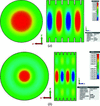Figure 3 Electric field patterns of the (a) HE11 and (b) HE12 modes.

Parameter scans were used to study the effects of varying of the corrugation geometry parameters such as the corrugation period (p), the slot length (w) and the waveguide radius (r1). The results of resonance frequency, Q factor and equivalent magnetic field strength are shown in Figs. 4–6. The HE11 mode has a similar trend to the HE12 mode. In Fig. 4, as the corrugation period increases, the resonance frequency becomes smaller while the slot length (w) and the waveguide radius (r1) are kept the same. The results matched the theoretical calculation well from equation (10) and the scaling law equations (Shumail et al., 2011). The difference between the simulation and theoretical calculation is less than 2%, therefore it allows a fast prediction of the cavity parameters from the theoretical analysis. The Q factor reduces as the corrugation period increases. It is mainly caused by the increase of the overall waveguide length and the decreases in the stored energy per unit length.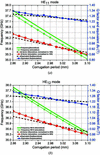Figure 4 Simulation results of different corrugation periods: (a) HE11 mode and (b) HE12 mode.

The simulation results at the different waveguide radii are shown in Fig. 5. The trends for the HE11 and HE12 modes are very similar. Increasing the waveguide radius would significantly increase the Q factor and the resonance frequency drops quickly. However, from equation (4), a high Q factor would result in a long filling time. For the HE11 mode, a 20% increment of the waveguide radius would lead to a Q factor close to 150 000, requiring an increase in the pulse length of the high-power microwave source used to drive the structure. Also, increasing the waveguide radius did not improve the equivalent magnetic field, which was almost the same. Therefore, the designed waveguide radius was considered to be optimal.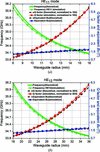Figure 5 Simulation results of different waveguide radii: (a) HE11 mode and (b) HE12 mode.

The simulation results of the variation in slot length while keeping the corrugation period constant are shown in Fig. 6. Only the CST simulation results were included as the theoretical analysis will not be accurate at small slot length values. As the slot length increases, the assumption of only the lowest TM standing wave appearing in the slot is no longer satisfied. The field at the corrugation wall became larger and caused a significant reduction in the cavity Q. The Q factor dropped more quickly for the HE11 mode than the HE12 mode because its waveguide radius was smaller. As the corrugation period was kept constant, the change in the resonance frequency was small. The equivalent magnetic field Bu also changed by a small amount. The simulation results proved that the slot length should be as small as possible to achieve a high Q factor. However, the difficulty in manufacturing such a structure while maintaining sufficient mechanical strength needs to be taken into account. A slot length of 0.5 mm is a reasonable value to satisfy the criteria of ease of manufacture, while at the same time being mechanically strong.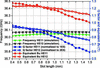Figure 6 Simulation results of different slot lengths.

### 5. Discussion and conclusions

In this paper, a corrugated waveguide was studied as a microwave undulator for a UK XFEL. The equations that govern the performance of the microwave undulator are presented and have been used to estimate the dimensions of the corrugated waveguide.

Both of the HE11 and HE12 modes existing in the corrugated waveguide are suitable operating modes. Their characteristics were studied using numerical simulation and their performance compared in the design of a corrugated waveguide operating at 36 GHz. It was found that the equivalent magnetic field for both of the modes was very similar. The HE12 mode had a much larger waveguide radius, which could be advantageous for high-frequency operation because it helps to reduce the difficulty in manufacture as well as reducing the electric field at the wall. The HE12 mode is also less sensitive to the slot length compared with the HE11 mode. The drawback of the HE12 mode includes a much higher Q factor that leads to a longer filling time and greater sensitivity to the waveguide period.

### Funding information

The authors would like to thank the Science and Technology Facilities Council (STFC) UK and the Cockcroft Institute (Core Grant ST/P002056/1) for supporting this work.

### References

This is an open-access article distributed under the terms of the Creative Commons Attribution (CC-BY) Licence, which permits unrestricted use, distribution, and reproduction in any medium, provided the original authors and source are cited.JOURNAL OFSYNCHROTRONRADIATION
ISSN: 1600-5775
Volume 26| Part 1| January 2019| Pages 11-17